#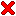Rigid Body Collision Resolutioninstantaneous collision resolution

This page tackles the problem of how to resolve the collision of two rigid bodies. My first encounter with this question was while designing a computer game in 2004. For over three years, I did not come across a satisfactory treatment neither in books, nor in the Internet. During my semester in Kiruna, I finally had the opportunity to thoroughly investigate the problem myself.

The input to the problem are:

• Initial states (0. and 1. order) of the bodies at the moment of contact,
• point, and normal of contact.

Without loss of generality, we assume:

• The center of total mass is at position 0, and
• the total linear momentum (impulse) is the null vector.

We are interested in

• resolving the collision within a single instance of time
• the velocity, and the angular velocity after the moment of contact.

We derive a mathematical solution to the problem step by step. To perform simulations and to visualize the objects in motion, we utilize a computer program, which is available for download. The implementation uses double precision, and simple Euler integration to solve the differential equations of rotation.

 Rigid Body Collision Resolution (C++) *ribocore.zip 230 kB Download this article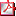rigid_body_collis... 320 kB
* The program checks the math, and produces the animations on this website.
It might be the warriors who get the glory,
but it's the engineers who build societies.
B'Elanna Torres

###Towards a mathematical solution

This section suggests forces and torques that - when applied to the bodies during collision - will preserve total linear momentum as well as total angular momentum. In this model, we allow the bodies to intrude each other. However, the forces and torques applied during collision make the bodies separate again.

In the illustrations, the ellipsoids visualize the inertia tensors of the bodies. The ellipsoids are not identical to the exterior of the body.

•billiard balls
•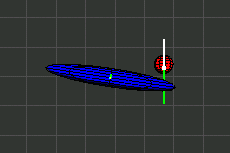baseball
•stuntmen

Most likely, the following notions are familiar to the reader.

The rigid bodies are enumerated by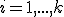. Each body has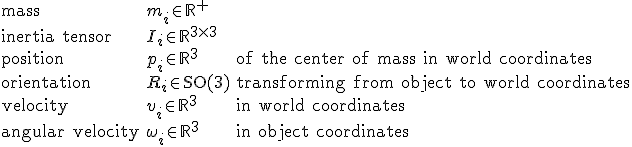Recall the assumptions stated above. The center of total mass is at position 0, i.e.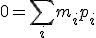and the total linear momentum (impulse) is the null vector: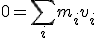We treat the collection of bodies as a closed system. Within this system, the total linear momentum, and the total angular momentum are perserved, i.e. constant vectors, at all times.

The total linear momentum is the sum of the linear momentums of each body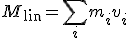The total angular momentum is the sum of the angular momentums of each body with respect to the center of total massSince in our world, the total momentums are constant, the derivative with respect to time is the null vector. I.e. we demand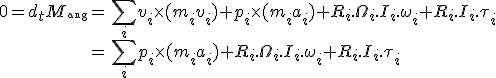In the above derivation, we assume that all quantities depend on time, except mass and the inertia tensors. The introduced variables represent the following: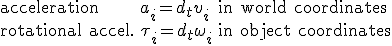whileis the angular velocity in matrix form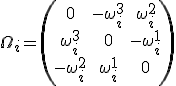We are interested in forces and torques applied to the bodies during the period of contact.

We specialize the formulas on two rigid bodies, i.e. we assume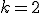. We introduce a few more symbols that characterize the contact between the two bodies:If bodies are intruding each other, then letbe proportional to the distance of intrusion. If the bodies are not in contact, we set. We suggest to apply the following forces and torquesWe show that under these conditions both, the total linear momentum and the total angular momentum are preserved. Conservation of total linear momentum follows because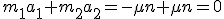The total angular momentum does not change becauseThese formulas produce the animations shown earlier.

Man muss wissen, bis wohin man zu weit gehen kann.
Jean Cocteau

###There is no time for procrastination.

In the introduction, we have stated the ultimate goal of this project: Given the linear and angular velocities just prior to the contact, we would like to have a formula that computes the linear and angular velocities just after the contact. This section suggests a solution to this problem. The solution is consistent with the definitions and derivations carried out earlier.

In the illustrations, the ellipsoids visualize the inertia tensors of the bodies. The ellipsoids are not identical to the exterior of the body.

•billiard balls
•baseball
•stuntmen

I hope you enjoy the upcoming formulas as much as I do.

First, we introduce a few more symbols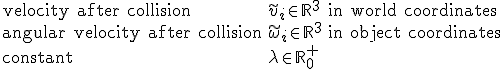We suggest: The linear and angular velocities just prior to the contact relate to the linear and angular velocities just after the contact in the following wayWe show that under these conditions both, the total linear momentum and the total angular momentum are preserved. Conservation of total linear momentum follows becauseThe total angular momentum after collision is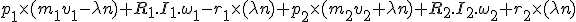From that, we subtract the total angular momentum before collisionand yield a difference ofAt this point,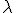is the only remaining unknown. As we will show next,is uniquely determined under the assumption that the two bodies collide elastically.

In an elastic collision, the total energy before the collision equals the total energy after the collision, i.e.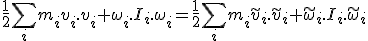Previously, we have suggested the relations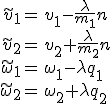whereNow, determiningso that the total energy is preserved reduces to solve a quadratic polynomial. Evidently,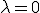is one solution to conserve total energy, which corresponds to "no collision". However, we are interested in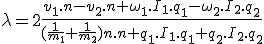Note, the numerator of the fraction is the scalar product between the velocity of contact and the surface normal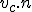, where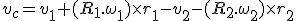Basically, we are done at this point. The latter value ofgenerates the three animations you see above. We conclude with some remarks:

• The forces and torques stated in the first section produce the same outcome if the collision process is modelled stiffly enough.
• Taking a fraction (for instance 95%) ofwill damp the collision, which might result in more realistic animations. Different fractions may correspond to different (non rigid) materials. The total linear momentum as well as the total angular momentum are preserved nevertheless.
• More general, both momentums are conserved for any vector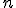, which is a convenient gateway for adapting the formula to less-elastic collisions.

To produce a final demonstration, we use the collision detection library RAPID. The white spheres indicate the location of last contact.

Wenn du Zeit gewinnen willst,
musst du Zeit verlieren.
Jean-Jacques Rousseau

###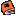Software and further reading

An open source physics engine that I have checked out myself, in the Newton Game Dynamics by Julio Jerez and Alain Suero. The software comes with a demo that explores the broad spectrum of applications. I find the results to be efficient, stable, and realistic. Moreover, the authors C++ style is just perfect.

The Open Dynamics Engine (ODE) originally by Russell Smith seems to be a popular C++ library for developers in gaming, but also in robotics. The library is suitable for simulating articulated rigid body structures. However, ODE emphasizes speed and stability over physical accuracy.

Computer graphics researchers from Stanford University came up with a successful strategy to animate and collide innumerable rigid bodies. The paper Nonconvex Rigid Bodies with Stacking describes how to achieve breathtaking results. An open source implementation of their method is written by Danny Chapman. To resolve a multi-contact multi-body collision, the authors propose to simulate the scenario a number of times: The sequence of pairwise collisions are resolved with different ordering and the outcomes are averaged.